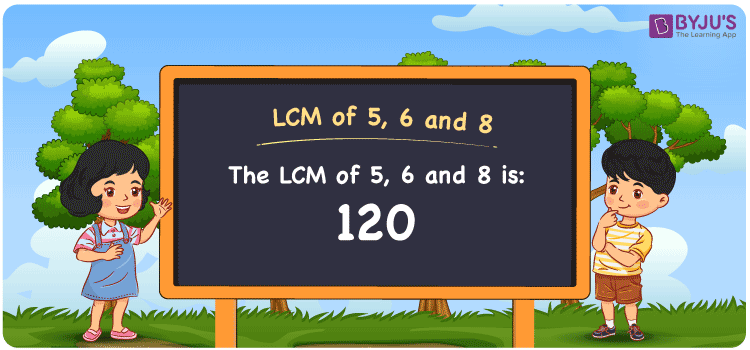# LCM of 5, 6 and 8

LCM of 5, 6 and 8 is 120. In Mathematics, the LCM is the value evenly divisible by the numbers given. The Least common multiple of 5, 6 and 8 is the smallest number calculated from the common multiples. (5, 10, 15, 20, 25, ….), (6, 12, 18, 24, 30, ….) and (8, 16, 24, 32, 40,….) are the multiples of 5, 6 and 8. We can find the LCM effortlessly using the prime factorisation method, division method and by listing the multiples.

Also read: Least common multiple

## What is LCM of 5, 6 and 8?

The answer to this question is 120. The LCM of 5, 6 and 8 using various methods is shown in this article for your reference. The LCM of two non-zero integers, 5, 6 and 8, is the smallest positive integer 120 which is divisible by both 5, 6 and 8 with no remainder.## How to Find LCM of 5, 6 and 8?

LCM of 5, 6 and 8 can be found using three methods:

• Prime Factorisation
• Division method
• Listing the multiples

### LCM of 5, 6 and 8 Using Prime Factorisation Method

The prime factorisation of 5, 6 and 8, respectively, is given by:

5 = 5¹

6 = 2 x 3 = 2¹ x 3¹

8 = 2 x 2 x 2 = 2³

LCM (5, 6, 8) = 120

### LCM of 5, 6 and 8 Using Division Method

We’ll divide the numbers (5, 6, 8) by their prime factors to get the LCM of 5, 6 and 8 using the division method (preferably common). The LCM of 5, 6 and 8 is calculated by multiplying these divisors.

 2 5 6 8 2 5 3 4 2 5 3 2 3 5 3 1 5 5 1 1 x 1 1 1

No further division can be done.

Hence, LCM (5, 6, 8) = 120

### LCM of 5, 6 and 8 Using Listing the Multiples

To calculate the LCM of 5, 6 and 8 by listing out the common multiples, list the multiples as shown below

• Multiples of 5 = 5, 10, 15, 20, 25, 30, 35, 40, 45, 50, . . . ., 110, 115, 120, . . . .
• Multiples of 6 = 6, 12, 18, 24, 30, 36, 42, 48, 54, 60, . . . ., 96, 102, 108, 114, 120, . . . .
• Multiples of 8 = 8, 16, 24, 32, 40, 48, 56, 64, 72, 80, . . . ., 96, 104, 112, 120, . . . .

The smallest common multiple of 5, 6 and 8 is 120.

Therefore LCM (5, 6, 8) = 120

## Video Lesson on Applications of LCM## LCM of 5, 6 and 8 Solved Examples

Question: In 20, 350, 120, 280, what is the LCM of 5, 6 and 8?

Solution:

The LCM value is the smallest number which is divisible exactly by 5, 6 and 8

The number 120 satisfies this condition

Hence, the LCM is 120.

## Frequently Asked Questions on LCM of 5, 6 and 8

### What is the LCM of 5, 6 and 8?

The LCM of 5, 6 and 8 is 120.

### Mention the methods used to determine the LCM of 5, 6 and 8.

The methods used to determine the LCM of 5, 6 and 8 are Prime Factorization Method, Division Method and Listing multiples.

### Calculate the GCF if the LCM of 5, 6 and 8 is 120.

LCM x GCF = 5 x 6 x 8

Given

LCM of 5, 6 and 8 = 120

120 x GCF = 240

GCF = 240/120 = 2

### Write the relation between GCF and LCM of 5, 6 and 8.

The relation between GCF and LCM of 5, 6 and 8 is

GCF x LCM = 5 x 6 x 8

GCF x LCM = 240

### Find the LCM of 5, 6 and 8 using prime factorisation.

Using prime factorisation

5 = 5¹

6 = 2 x 3 = 2¹ x 3¹

8 = 2 x 2 x 2 = 2³

LCM of 5, 6 and 8 is 120.